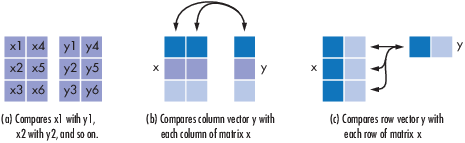symerr

Compute number of symbol errors and symbol error rate

Description

example

[number,ratio] = symerr(x,y) compares the elements in x and y. The sizes of x and y determine which elements are compared. The output number is a scalar or vector that indicates the number of elements that differ. The output ratio equals number divided by the total number of elements in the smaller input.

[number,ratio] = symerr(x,y,flg) compares the elements in x and y. Optional input flg and the size of x, and y, determine the size of number.

example

[number,ratio,individual] = symerr(...) returns a binary matrix individual that indicates which elements of x and y differ. An element of individual is zero if the corresponding comparison yields no discrepancy, and one otherwise.

Examples

collapse all

Compare Elements of Matrix with Another Matrix

x = [1,1,3,1;3,2,2,2;3,3,8,3]
x = 3×4

1     1     3     1
3     2     2     2
3     3     8     3

aMatrix = [1,1,1,1;2,2,2,2;3,3,3,3]
aMatrix = 3×4

1     1     1     1
2     2     2     2
3     3     3     3

[number1,ratio1] = symerr(x,aMatrix)
number1 = 3
ratio1 = 0.2500

Compare Elements of Matrix with Row Vector

x = [1,1,3,1;3,2,2,2;3,3,8,3]
x = 3×4

1     1     3     1
3     2     2     2
3     3     8     3

aRowVector = [1,2,3,1]
aRowVector = 1×4

1     2     3     1

[number2,ratio2] = symerr(x,aRowVector)
number2 = 3×1

1
3
4

ratio2 = 3×1

0.2500
0.7500
1.0000

Compare Elements of Matrix with Column Vector

x = [1,1,3,1;3,2,2,2;3,3,8,3]
x = 3×4

1     1     3     1
3     2     2     2
3     3     8     3

aColumnVector = [1;2;3]
aColumnVector = 3×1

1
2
3

[number3,ratio3] = symerr(x,aColumnVector)
number3 = 1×4

1     0     2     0

ratio3 = 1×4

0.3333         0    0.6667         0

You can specify alternative comparison methods used by symerr. In this example, you use a flag to override the default row-by-row comparison. Notice that number and ratio are scalars.

format rat;
[number,ratio,loc] = symerr([1 2; 3 4],[1 3],'overall')
number =
3

ratio =
3/4

loc =
0              1
1              1

Input Arguments

collapse all

First input to compare, specified as a vector, or a matrix.

Data Types: double

Second input to compare, specified as a vector, or a matrix.

Data Types: double

Optional argument to override the defaults that govern which elements symerr compares and how symerr computes the outputs.

• 'overall' –– x and y are compared element by element.

• 'column-wise' –– mth row of x vs. mth row of y.

• 'row-wise' –– mth column of x vs. mth column of y.

Output Arguments

collapse all

Number of elements that differ between x and y, returned as a scalar or vector. The size of number is determined by the optional input flg and by the dimensions of x and y. For more information, see the Default Element Comparison and Specifying Element Comparison sections.

The ratio of the number of differing elements, number, and the total number of elements of the smaller input, returned as a scalar.

Results of each individual symbol comparison, returned as a matrix of same size and dimensions as inputs x and y. The output matrix contains zeros for all locations corresponding to the elements of x and y that are equal, and ones where the two elements differ.

Data Types: logical

collapse all

Default Element Comparison

The symerr function compares binary representations of elements in x with those in y. When optional argument flg is not specified, symerr uses the shape of the inputs x and y to determine the element comparison method.

The schematics below illustrate how the shapes of x and y determine which elements symerr compares:• If x and y are matrices of the same dimensions, then symerr compares x and y element by element. number is a scalar. See schematic (a) in the figure.

• If one is a row (respectively, column) vector and the other is a two-dimensional matrix, then symerr compares the vector element by element with each row (resp., column) of the matrix. The length of the vector must equal the number of columns (resp., rows) in the matrix. number is a column (resp., row) vector whose mth entry indicates the number of elements that differ when comparing the vector with the mth row (resp., column) of the matrix. See schematics (b) and (c) in the figure.

Specifying Element Comparison

Use flg to override the defaults that govern which elements symerr compares and how symerr computes the outputs. The values of flg are 'overall', 'column-wise', and 'row-wise'. The table below describes the differences that result from various combinations of inputs. In all cases, ratio is number divided by the total number of elements in y.

Comparing a Two-Dimensional Matrix x with Another Input y

Shape of yflgType of Comparisonnumber
Two-dim. matrix 'overall' (default) Element by element Total number of symbol errors
'column-wise'mth column of x vs. mth column of y Row vector whose entries count symbol errors in each column
'row-wise'mth row of x vs. mth row of y Column vector whose entries count symbol errors in each row
Column vector 'overall' y vs. each column of x Total number of symbol errors
'column-wise' (default) y vs. each column of x Row vector whose entries count symbol errors in each column of x

Row vector

'overall'y vs. each row of x Total number of symbol errors
'row-wise' (default) y vs. each row of x Column vector whose entries count symbol errors in each row of x